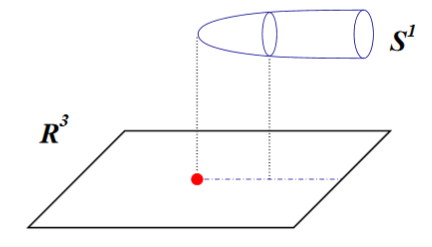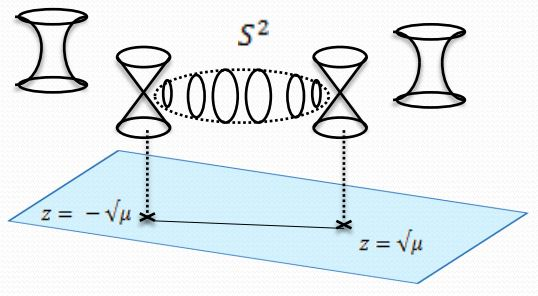# nLab Kaluza-Klein monopole

Contents

### Context

#### Gravity

gravity, supergravity

## Quantum theory

#### Riemannian geometry

Riemannian geometry

# Contents

## Idea

### General

In the context of Kaluza-Klein theory where an electromagnetic field in Einstein-Maxwell theory in dimension $d$ is modeled by a configuration of pure Einstein gravity in dimension $d+1$, a Kaluza-Klein monopole is a configuration of gravity in dimension $d+1$ which in dimension $d$ looks like a magnetic monopole (Sorkin 83, Gross-Perry 83).

### In supergravity

This situation is of particular interest in the reduction of 11-dimensional supergravity (or M-theory, where one also speaks of the MK6-brane) where the Kaluza-Klein magnetic monopole charge is interpreted as D6-brane charge under duality between M-theory and type IIA string theory.

The Kaluza-Klein monopole (Han-Koh 85) is one type of solution of the equations of motion of 11-dimensional supergravity. It is given by the product $N_4\times \mathbb{R}^{11-5,1}$ of Euclidean Taub-NUT spacetime with Minkowski spacetime. Upon Kaluza-Klein compactification this looks like a monopole, whence the name. (For discussion as an ADE-singularity see IMSY 98, section 9, Asano 00, section 3.)

Upon KK-compactification on a 6-dimensional fiber, with the 11d KK-monopole / D6-brane completely wrapping the fiber, the KK-monopole in 11d supergravity becomes the KK-monopole in 5d supergravity. Further compactifying on a circle leads to a black hole in 4d, incarnated as a D0/D6 bound state (e.g. Nelson 93).

geometry transverse to KK-monopolesRiemannian metricremarks
Taub-NUT space:
geometry transverse to
$N+1$ distinct KK-monopoles
at $\vec r_i \in \mathbb{R}^3 \;\; i \in \{1, \cdots, N+1\}$
$\array{d s^2_{TaubNUT} \coloneqq U^{-1}(d x^4 + \vec \omega \cdot d \vec r)^2 + U (d \vec r)^2 \,, \\ \vec r \in \mathbb{R}^3,\, x^4 \in \mathbb{R}/(2 \pi R\mathbb{Z}) \\ U \coloneqq 1 + \underoverset{i = 1}{N+1}{\sum} U_i\,, \phantom{AA} \vec \omega \coloneqq \underoverset{i = 1}{N+1}{\sum} \vec \omega_i \\ U_i \coloneqq \frac{R/2}{ {\vert \vec r - \vec r_i\vert} }\,, \phantom{AA} \vec \nabla \times \vec \omega= \vec \nabla U_i}$(e.g. Sen 97b, Sect. 2)
ALE space
Taub-NUT close to $N$ close-by KK-monopoles
e.g. close to $\vec r = 0$: $\frac{{\vert \vec r_i\vert}}{R/2}, \frac{{\vert \vec r\vert}}{R/2} \ll 1$
$\array{d s^2_{ALE} \coloneqq U'^{-1}(d x^4 + \vec \omega \cdot d \vec r)^2 + U' (d \vec r)^2 \,, \\ \vec r \in \mathbb{R}^3,\, x^4 \in \mathbb{R}/(2 \pi R\mathbb{Z}) \\ U' \coloneqq \underoverset{i = 1}{N+1}{\sum} U'_i\,, \phantom{AA} \vec \omega \coloneqq \underoverset{i = 1}{N+1}{\sum} \vec \omega_i \\ U'_i \coloneqq \frac{R/2}{ {\vert \vec r - \vec r_i\vert} }\,, \phantom{AA} \vec \nabla \times \vec \omega= \vec \nabla U_i}$e.g. via Euler angles: $\vec \omega = (N+1)R/2(\cos(\theta)-1) d\psi$
(e.g. Asano 00, Sect. 2)
$A_N$-type ADE singularity:
ALE space in the limit
where all $N+1$ KK-monopoles coincide at $vec r_i = 0$
$\array{d s^2_{A_N Sing} \coloneqq \frac{\vert\vec r\vert }{(N+1)R/2}(d x^4 + \vec \omega \cdot d \vec r)^2 + \frac{ (N+1)R/2}{\vert \vec r\vert} (d \vec r)^2 \,, \\ \vec r \in \mathbb{R}^3,\, x^4 \in \mathbb{R}/(2 \pi R\mathbb{Z}) }$(e.g. Asano 00, Sect. 3)

## Properties

(For the moment the following is all about the KK-monopoles in 11d supergravity/M-theory.)

### Far-horizon geometry

We discuss the far horizon geometry of coincident MK6-branes.

The metric tensor of $N$ coincident KK-monopoles in 11-dimensional supergravity in the limit that $\ell_{th} \coloneqq N \ell_P \to 0$ is

(1)$g_{MK6} \;=\; g_{\mathbb{R}^{6,1}} + (d y)^2 + y^2 \big( (d \theta)^2 + (\sin \theta)^2 (d \varphi)^2 + (\cos \theta)^2 (d \phi)^2 \big)$

subject to the identification

(2)$(\varphi, \phi) \;\sim\; (\varphi, \phi) + (2\pi/N ,2\pi/N) \,.$

This is equation (47) in IMSY 98, which applies subject to the condition

$U/\left(\frac{N}{g^{2/3}_{YM}}\right) \;=\; U/\left(\frac{N}{(2\pi)^{4/3} \ell_P}\right) \;\gg\; 1$

from a few lines above. Inserting this condition into the definition $y^2 \coloneqq 2 N \ell^3_P U$ right above (47) shows that

\begin{aligned} y^2 & = 2 N \ell^3_P U \\ & = 2(2\pi)^{-4/3} N^2 \ell_P^2 \; \underset{ \gg 1 }{ \underbrace{ \left(U/\left(\frac{N}{ (2 \pi)^{4/3} \ell_P}\right)\right) }} \end{aligned}

hence that the distance $y$ from the locus of the MK6-brane is large in units of

$\ell_{th} \;=\; \sqrt{2} (2\pi)^{-2/3} N \ell_P \,.$

The identification (2) means that this is the orbifold metric cone $\mathbb{R}^{6,1} \times \left( \mathbb{R}^4/(\mathbb{Z}_N)\right)$, hence an A-type ADE-singularity. To make this more explicit, introduce the complex coordinates

$v \;\coloneqq\; y \, e^{i \varphi} \sin \theta \;\;\; w \;\coloneqq\; y \, e^{i \phi} \cos \theta$

on $\mathbb{R}^4 \simeq \mathbb{C}^2$, in terms of which (1) becomes

$g_{MK6} \;\coloneqq\; d v d \overline v + d w d \overline w$

and which exhibit the identification (2) as indeed that of the A-type $\mathbb{Z}_N$-action (Asano 00, around (18)).

### Relation to the D6-brane in type IIA string theory

Under the relation between M-theory and type IIA superstring theory an ADE orbifold of the 11d KK-monopole corresponds to D6-branes combined with O6-planes (Townsend 95, p. 6, Atiyah-Witten 01, p. 17-18 see also e.g. Berglund-Brandhuber 02, around p. 15).

By (Townsend 95, (1), Sen 97c (1)-(4)) the 11d spacetime describing the KK-monopole lift of a plain single D6 brane is $\mathbb{R}^{6,1}\times \mathbb{R}^3\times S^1$ with metric tensor away from the origin of the $\mathbb{R}^3$-factor (which is the locus of the lifted D6/monopole) being

$d s_{11}^2 = - d t^2 + d s_{\mathbb{R}^6}^2 + (1+\mu/r) d s_{\mathbb{R}^3}^2 + (1+ \mu/r)^{-1} (d x^{11} - A_i d x^i)^2 \,,$

where

• $A = A_i d x^i$ is any 3-form on $\mathbb{R}^3$ satisfying $d_{\mathbb{R}^3} A = \star d (1-\mu/r)$;

• $r$ denotes the distance in $\mathbb{R}^3$ from the origin.

• $\mu$ is the charge of the monopole.Away from $\{0\} \in \mathbb{R}^3$ this gives a circle bundle with first Chern class measured by the integral of $R_0 \coloneqq d A$ (the RR-field of the D0-brane) over any sphere surrounding the singular locus in $\mathbb{R}^3 - \{0\}$. By the electric-magnetic duality between D0-branes and D6-branes (due to the self-duality of the RR-field) this means, from the 10-dimensional perspective, that at $0 \in \mathbb{R}^3$ a D6-brane is located.

graphics grabbed from Acharya-Gukov 04

Notice that the circle bundle away from its degeneration locus as a bundle over $S^2 \hookrightarrow \mathbb{R}^3 -\{0\}$ is necessarily of the form $S^3 \to S^2$, a multiple of the complex Hopf fibration (see also Atiyah-Maldacena-Vafa 00, p. 10).

Discussion as an A-type ADE singularity is in (Sen 97c, section 2). Generalization to D-type singularities and hence D6-branes in orientifolds is in (Sen 97c ,section 3). Discussion as the fixed point of the circle group-action on the M-theory circle fibers is in (Townsend 95, p. 6, Atiyah-Witten 01, pages 17-18). Witten emphasizes that it is important that the location of the D6 is not just a cyclic group orbifold singularity but really a circle group-action fixed point conical singularity:

Chiral fermions arise when the locus of A−D−E singularities passes through isolated points at which X has an isolated conical singularity that is not just an orbifold singularity (Witten 01, p. 2).

Discussion dealing carefully with the perspective where the locus of the D6-brane is not taken out is in (Gaillard-Schmude 09).

For more on this see at D6-brane – Relation to other branes and at M-theory lift of gauge enhancement on D6-branes.

### Relation to the D7-brane in type IIB string theory/F-theory

Under further T-duality to type IIB superstring theory/F-theory these D6-branes become the D7-branes.

from M-branes to F-branes: superstrings, D-branes and NS5-branes

M-theory on $S^1_A \times S^1_B$-elliptic fibrationKK-compactification on $S^1_A$type IIA string theoryT-dual KK-compactification on $S^1_B$type IIB string theorygeometrize the axio-dilatonF-theory on elliptically fibered-K3 fibrationduality between F-theory and heterotic string theoryheterotic string theory on elliptic fibration
M2-brane wrapping $S_A^1$double dimensional reduction $\mapsto$type IIA superstring$\mapsto$type IIB superstring$\mapsto$$\mapsto$heterotic superstring
M2-brane wrapping $S_B^1$$\mapsto$D2-brane$\mapsto$D1-brane$\mapsto$
M2-brane wrapping $p$ times around $S_A^1$ and $q$ times around $S_B^1$$\mapsto$$p$ strings and $q$ D2-branes$\mapsto$(p,q)-string$\mapsto$
M5-brane wrapping $S_A^1$double dimensional reduction $\mapsto$D4-brane$\mapsto$D5-brane$\mapsto$
M5-brane wrapping $S_B^1$$\mapsto$NS5-brane$\mapsto$NS5-brane$\mapsto$$\mapsto$NS5-brane
M5-brane wrapping $p$ times around $S_A^1$ and $q$ times around $S_B^1$$\mapsto$$p$ D4-brane and $q$ NS5-branes$\mapsto$(p,q)5-brane$\mapsto$
M5-brane wrapping $S_A^1 \times S_B^1$$\mapsto$$\mapsto$D3-brane$\mapsto$
KK-monopole/A-type ADE singularity (degeneration locus of $S^1_A$-circle fibration, Sen limit of $S^1_A \times S^1_B$ elliptic fibration)$\mapsto$D6-brane$\mapsto$D7-branes$\mapsto$A-type nodal curve cycle degeneration locus of elliptic fibration(Sen 97, section 2)SU-gauge enhancement
KK-monopole orientifold/D-type ADE singularity$\mapsto$D6-brane with O6-planes$\mapsto$D7-branes with O7-planes$\mapsto$D-type nodal curve cycle degeneration locus of elliptic fibration(Sen 97, section 3)SO-gauge enhancement
exceptional ADE-singularity$\mapsto$$\mapsto$$\mapsto$exceptional ADE-singularity of elliptic fibration$\mapsto$E6-, E7-, E8-gauge enhancement

(e.g. Johnson 97, Blumenhagen 10)

### Other Brane charges (?)

In (Hull 97) it was argued that the KK-monopole in 11-dimensional supergravity is the object which carries the 6-form charge Poincaré dual to the time-component of the 5-form charge of the M5-brane as appearing in the M-theory super Lie algebra via

$\wedge^5 (\mathbb{R}^{10,1})^\ast \simeq \wedge^5 (\mathbb{R}^{10})^\ast \oplus \wedge^6 \mathbb{R}^{10} \,.$

(The same kind of relation identifies the time-component of the M2-brane charge with the charge of the M9-brane, see there.)

### In 5d gravity

Original articles:

Review includes

• Emre Sakarya, Kaluza-Klein monopole, 2007 (pdf)

Discussion of topological T-duality for KK-monopoles is in

• Ashwin S. Pande, Topological T-duality and Kaluza-Klein Monopoles, Adv. Theor. Math. Phys., 12, (2007), pp 185-215 (arXiv:math-ph/0612034)

### In 11d supergravity

#### Original articles

Discussion in terms of G-structures:

Discussion of a BFSS-like matrix model for MK6-branes:

• Amihay Hanany, Gilad Lifschytz, M(atrix) Theory on $T^6$ and a m(atrix) Theory Description of KK Monopoles, Nucl. Phys. B519:195-213, 1998 (arXiv:hep-th/9708037)

### Relation to black holes

Relation to black holes in string theory

• William Nelson, Kaluza-Klein Black Holes in String Theory, Phys. Rev. D49:5302-5306, 1994 (arXiv:hep-th/9312058)